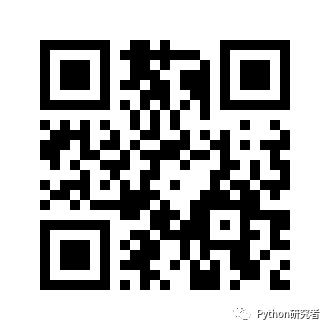﻿ Python实现动态二维码生成的示例代码_python_脚本之家
python# Python实现动态二维码生成的示例代码

## 1.MyQR

`pip install MyQR`

### 普通的二维码制作

```from MyQR import myqr
#运行
myqr.run('http://mtw.so/5w0Ubz')```### 带背景图片的二维码制作

```from MyQR import myqr
text = "http://mtw.so/5w0Ubz"
#给二维码添加图片背景
myqr.run(
text,
picture='./pythonyjz.jpg',
colorized=True,
version=8
)
```• words：二维码内容
• version：二维码大小，范围为 [1,40]
• picture：二维码背景图 .jpg，.png，.bmp，.gif，默认为黑白色
• colorized：二维码背景颜色，默认 False，即黑白色
• save_name：二维码名称，默认为 qrcode.png
• save_dir：二维码路径，默认为程序当前路径### 带动图背景的二维码制作

```from MyQR import myqr

text = "http://mtw.so/5w0Ubz"
#给二维码添加动图gif背景
myqr.run(
text,
#动图gif
picture='./pythonyjz.gif',
colorized=True,
version=8
)
```## 2.qrcode

```pip install qrcode
pip install Image
pip install matplotlib```

### 简单的二维码制作

```# 导入模块
import qrcode
# 传入将要生成二维码的URL
img = qrcode.make('http://mtw.so/5w0Ubz')
# 保存
img.save('python研究者-1.png')``````import qrcode
qr = qrcode.QRCode(
version=10,
# 二维码的纠错功能
error_correction=qrcode.constants.ERROR_CORRECT_L,
# 二维码中每个小格子包含的像素
box_size=10,
# 边框包含的格子数，默认为4
border=4,
)
# 添加数据
# 填充数据
qr.make(fit=True)
# 二维码的背景色和格子色
img=qr.make_image(fill_color='black', back_color='white');
img.save('python研究者-2.png')
```### 带背景图片的二维码制作

```from PIL import Image
import qrcode
import matplotlib.pyplot as plt
qr = qrcode.QRCode(
version=1,
error_correction=qrcode.constants.ERROR_CORRECT_H,
box_size=5,
border=4,
)

# 添加数据
# 填充数据
qr.make(fit=True)
# 生成图片
img = qr.make_image(fill_color="green", back_color="white")

# 添加logo，打开logo照片
icon = Image.open("pythonyjz.jpg")
# 获取图片的宽高
img_w, img_h = img.size
# 参数设置logo的大小
factor = 6
size_w = int(img_w / factor)
size_h = int(img_h / factor)
icon_w, icon_h = icon.size
if icon_w > size_w:
icon_w = size_w
if icon_h > size_h:
icon_h = size_h
# 重新设置logo的尺寸
icon = icon.resize((icon_w, icon_h), Image.ANTIALIAS)
# 得到画图的x，y坐标，居中显示
w = int((img_w - icon_w) / 2)
h = int((img_h - icon_h) / 2)
# 黏贴logo照
# 保存img
img.save('python研究者-3.png')
```